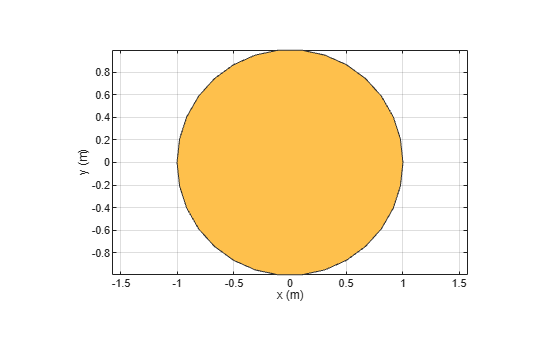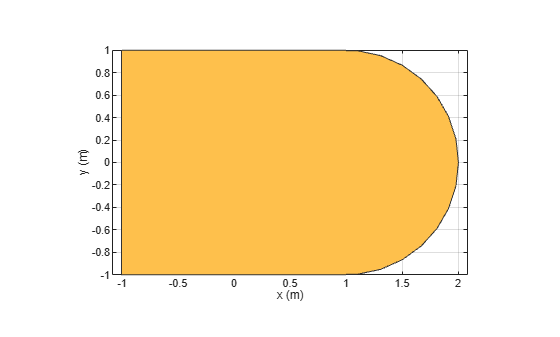# antenna.Circle

Create circle centered at origin on X-Y plane

## Description

Use the `antenna.Circle` object to create a circle centered at the origin and on the X-Y plane. You can use `antenna.Polygon` to create single-layer or multi-layered antennas using `pcbStack`.

## Creation

### Syntax

``circle = antenna.Circle``
``circle = antenna.Circle(Name,Value)``

### Description

example

````circle = antenna.Circle` creates a circle centered at the origin and on the X-Y plane.```

example

````circle = antenna.Circle(Name,Value)` sets properties using one or more name-value pair. For example, ```circle = antenna.Circle('Radius',0.2)``` creates a circle of radius 0.2 m. Enclose each property name in quotes.```

## Properties

expand all

Name of circle, specified a character vector.

Example: `'Name','Circle1'`

Example: `circle.Name= 'Circle1'`

Data Types: `char` | `string`

Cartesian coordinates of center of circle, specified a 2-element vector with each element measured in meters.

Example: `'Center',[0.006 0.006]`

Example: `circle.Center= [0.006 0.006]`

Data Types: `double`

Circle radius, specified a scalar in meters.

Example: `'Radius',2`

Example: `circle.Radius= 2`

Data Types: `double`

Number of discretization points on circumference, specified a scalar.

Example: `'NumPoints',16`

Example: `circle.NumPoints= 2`

Data Types: `double`

## Object Functions

 `add` Boolean unite operation on two shapes `subtract` Boolean subtraction operation on two shapes `area` Calculate area of shape in square meters `intersect` Boolean intersection operation on two shapes `rotate` Rotate shape about axis and angle `rotateX` Rotate shape about x-axis and angle `rotateY` Rotate shape about y-axis and angle `rotateZ` Rotate shape about z-axis and angle `translate` Move shape to new location `show` Display antenna or array structure; display shape as filled patch `mesh` Mesh properties of metal or dielectric antenna or array structure `removeHoles` Remove holes from shape `removeSlivers` Remove sliver outliers from boundary of shape

## Examples

collapse all

Create and view circle using `antenna.Circle` and view it.

`c1 = antenna.Circle`
```c1 = Circle with properties: Name: 'mycircle' Center: [0 0] Radius: 1 NumPoints: 30 ```
`show(c1)`Create a circle with a radius of 4 m.

`c2 = antenna.Circle('Radius',4)`
```c2 = Circle with properties: Name: 'mycircle' Center: [0 0] Radius: 4 NumPoints: 30 ```

Create circle with a radius of 1 m. The center of the circle is at [1 0].

`circle1 = antenna.Circle('Center',[1 0],'Radius',1);`

Create a rectangle with a length of 2 m and a width of 4 m centered at the origin.

`rect1 = antenna.Rectangle('Length',2,'Width',2);`

Add the two shapes together using the `+` function.

`polygon1 = circle1+rect1`
```polygon1 = Polygon with properties: Name: 'mypolygon' Vertices: [21x3 double] ```
`show(polygon1)`## Version History

Introduced in R2017a